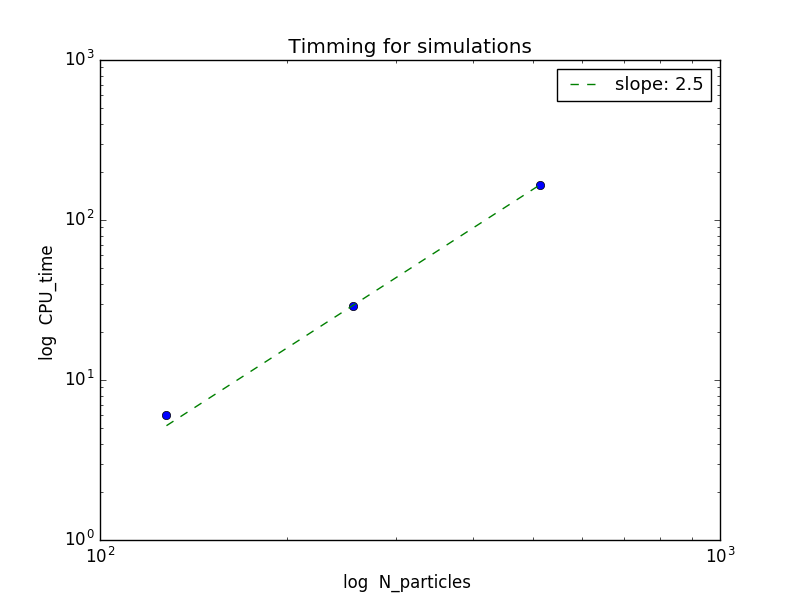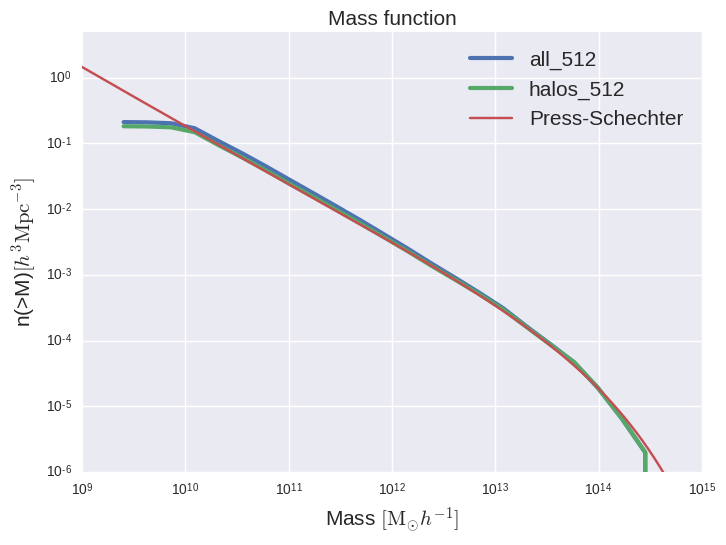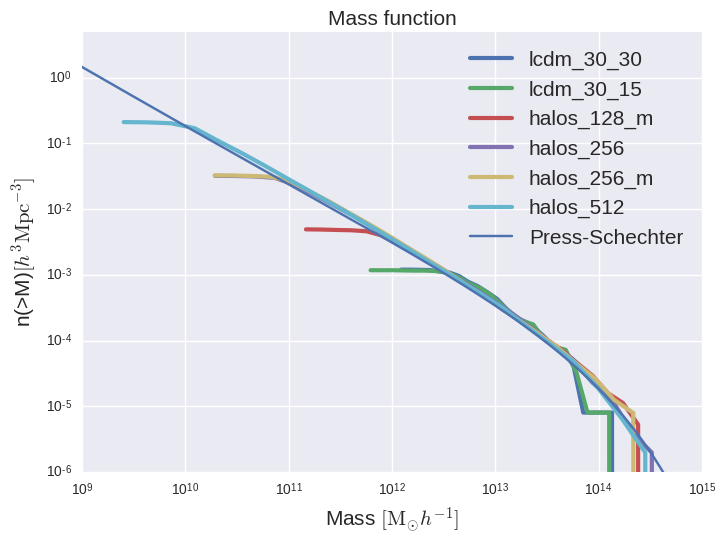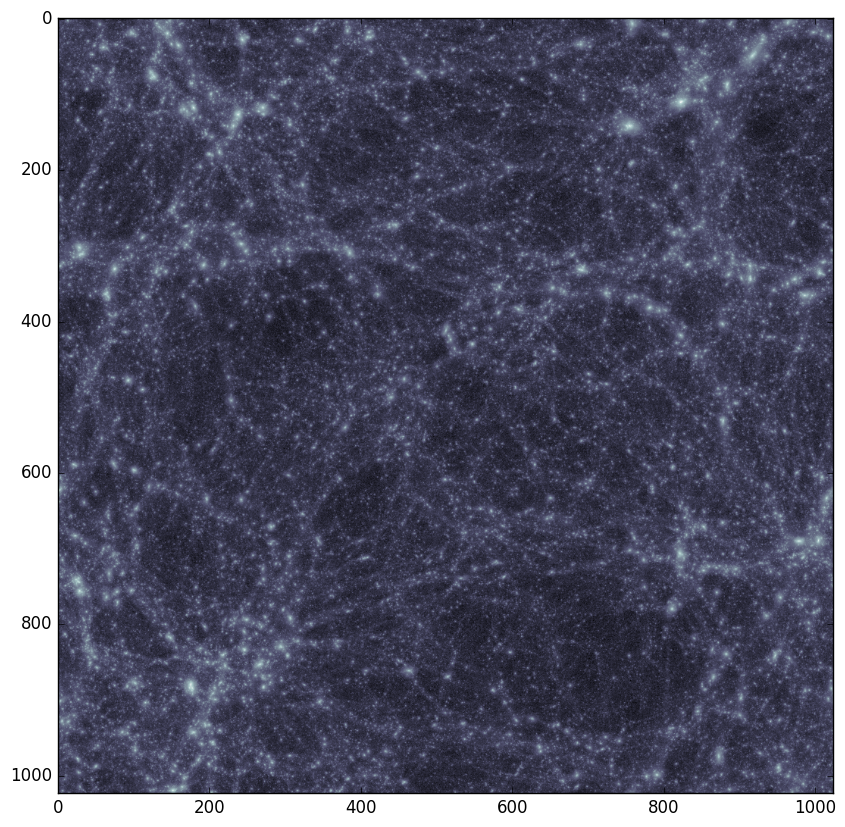Parameters used for Millenium simulations Here

The size of the box is 115 Mpc/h

$N_{part}$ $\epsilon$ [kpc/h] CPU Time [Hrs] Wall Time [Hrs]
$128^3$ 19.41 6.0 0.4
$256^3$ 9.70 29.0 1.8
$512^3$ 4.85 165.5 10.3
$1024^3$ 2.42
$2048^3$ 1.21
$4096^3$ 0.61

CPU_TIME as function of N_PARTICLES:Mass function from ROCKSTAR halo catalogs:Density projection for $512^3$ simulation using Brant’s code (image-snapshot):Error running $1024^3$ simulation HERE